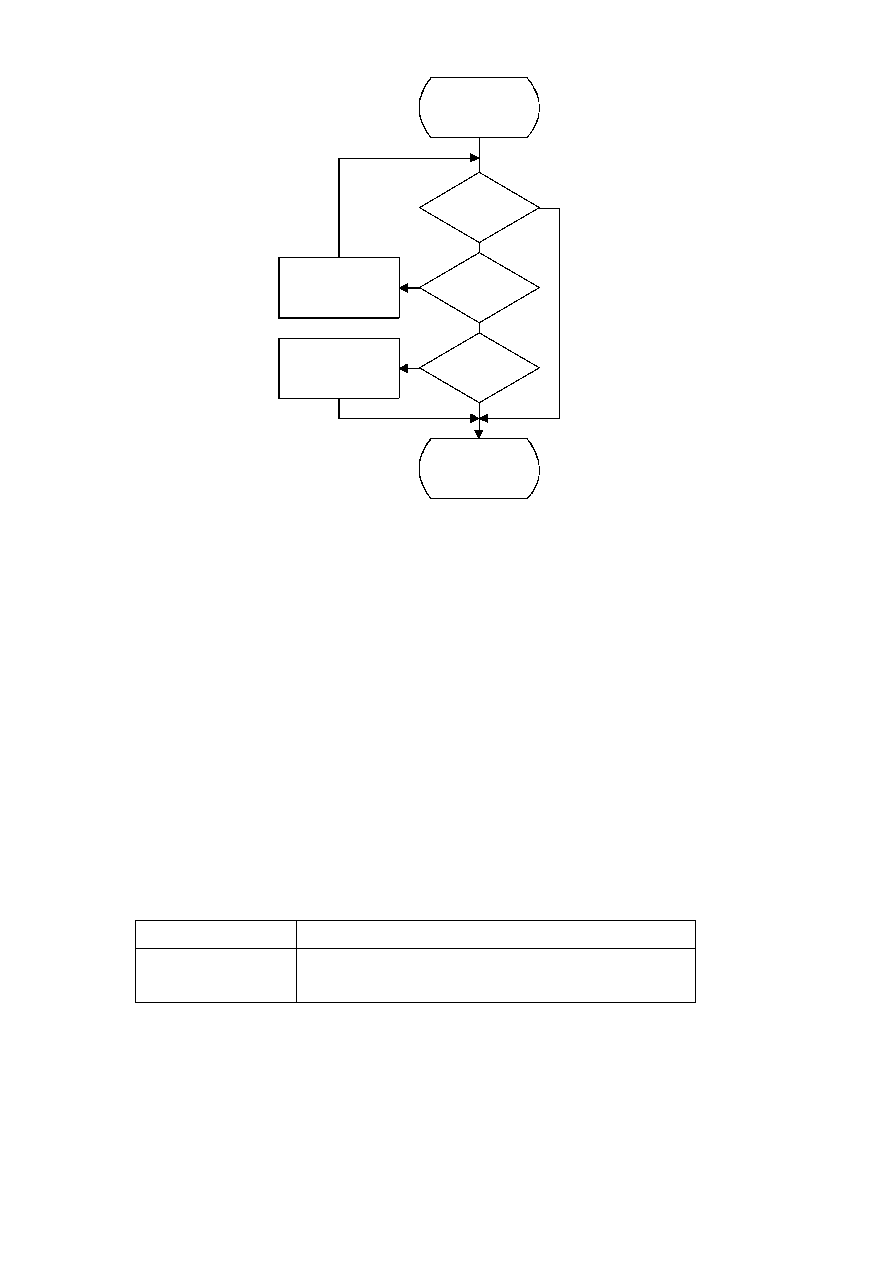ISO/IEC 10918-1 : 1993(E)
TISO1150-93/d053
BP = BP ­ 1
BP = BP + 1
Done
Yes
Yes
Yes
No
No
No
BP < BPST
?
B = 0
?
B = X'FF'
?
Figure D.15 ­ Discard_final_zeros procedure in Flush
Figure D.15 [D53], = 12.5cm = 489.%
D.2
Arithmetic decoding procedures
Two arithmetic decoding procedures are used for arithmetic decoding (see Table D.4).
The "Decode(S)" procedure decodes the binary decision for a given context-index S and returns a value of either 0 or 1. It
is the inverse of the "Code_0(S)" and "Code_1(S)" procedures described in D.1. "Initdec" initializes the arithmetic
coding entropy decoder.
Table D.4 ­ Procedures for binary arithmetic decoding
Procedure
Purpose
Decode(S)
Decode a binary decision with context-index S
Initdec
Initialize the decoder
CCITT Rec. T.81 (1992 E)
69